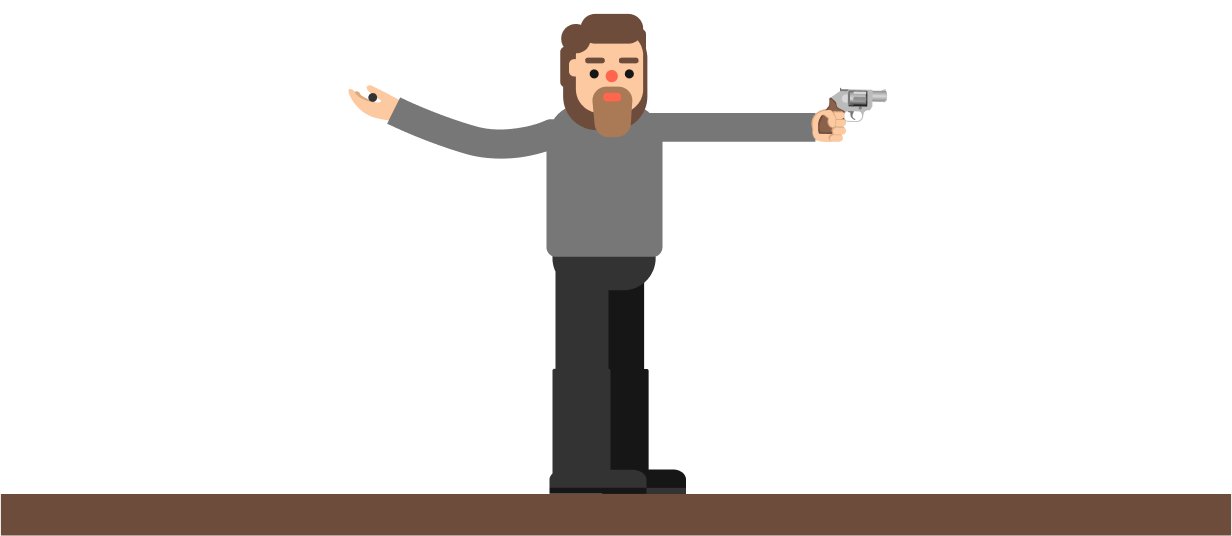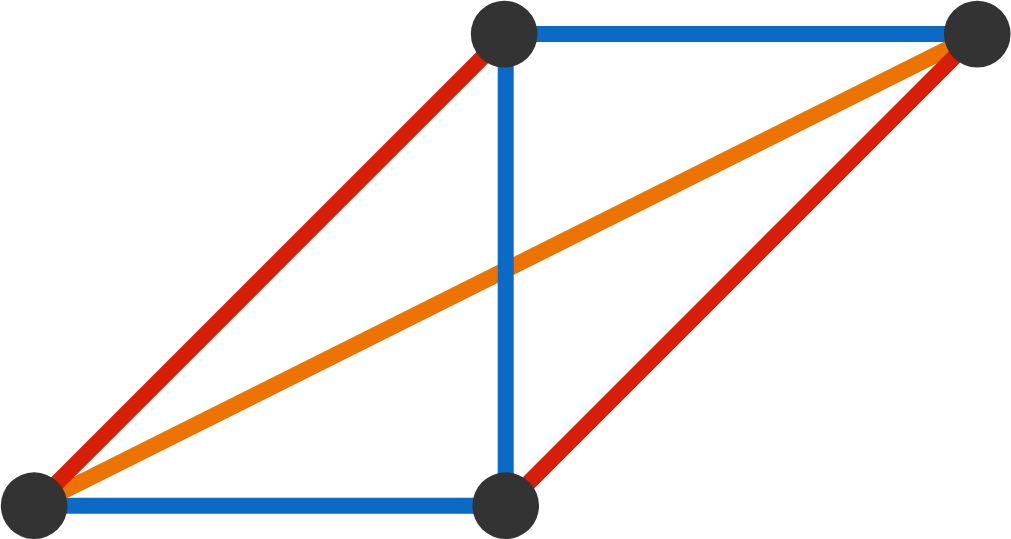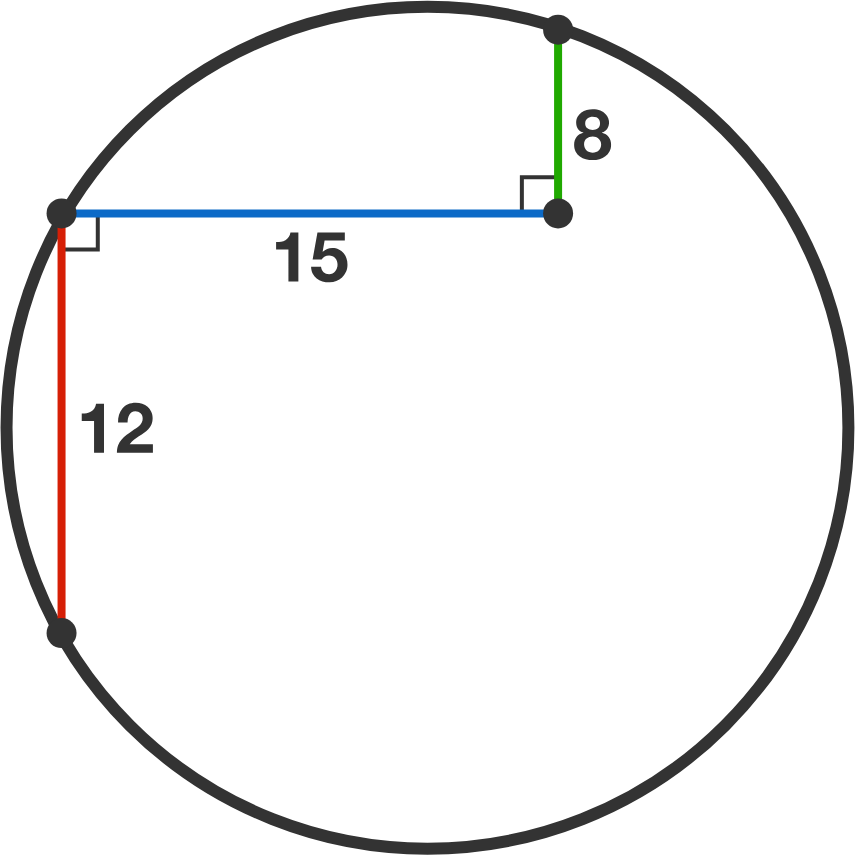# Problems of the Week

Contribute a problem

# 2018-09-03 Intermediate

A gun fires a spherical bullet parallel to a horizontal, flat plane. An identical bullet is dropped at the same time, from the same height.

Which bullet will hit the plane first?Hint: Compare the air resistance acting on the bullets.

The prime number $31$ can be expressed in the form $n^5-1$, where $n=2$.

Are there any other primes that can be expressed in the form $n^5 - 1,$ where $n$ is a positive integer?

Bonus: If the answer is yes, then what is the next number? If the answer is no, then why?This arrangement requires three different colors of string.

The diagram shows four black pins put on a board. Six colored strings connect each pair of pins, and the same-colored strings have the same length.

We would like to rearrange the four pins in such a way that we need exactly two different colors for the six connecting strings.

How many ways are there to rearrange the pins in this manner?

Note: The four pins must make a distinct shape for each solution. Rotations, reflections, and dilations are not considered new solutions.What is the radius of this circle?

Aaron has blood type $A$, Belle has blood type $B$, and their 4 children have blood types $O, A, B, AB$. This is possible because the genotypes of the parents are $AO$ and $BO$, and their children each receive one random genotype from each parent, resulting in genotypes $OO, AO, BO, AB$. See ABO blood group system for more info.

Aaron's family lives in Mathville. In the population, $32\text{\%}$ have blood type $A$ and $4\text{\%}$ have blood type $AB$. The ratio of blood type is stable, meaning the blood type ratios are expected to stay the same from generation to generation. Specifically, the probability a newborn has a certain blood type from 2 random individuals is equal to the population percentage of that blood type.

What is the percentage of population having blood type $O$ (rounded to the nearest integer)?

×

Problem Loading...

Note Loading...

Set Loading...